Main Content

# Create Image Comparison Tool Using ROIs

This example shows how to use the new ROI functions to create an interactive image comparison tool. When working with images, we often need to assess visually the impact a function has on an image. Some effects are clearly distinguishable, like an edge filter. But some impacts are more subtle and need closer inspection.

### Read Sample Image into the Workspace

Read a sample image into the workspace and then create a grayscale version of the image. Use the `imshowpair` function to view the two images. The `montage` option shows the images side-by-side.

```im = imread('peppers.png'); imgray = rgb2gray(im); figure; imshowpair(im,imgray,'montage') ```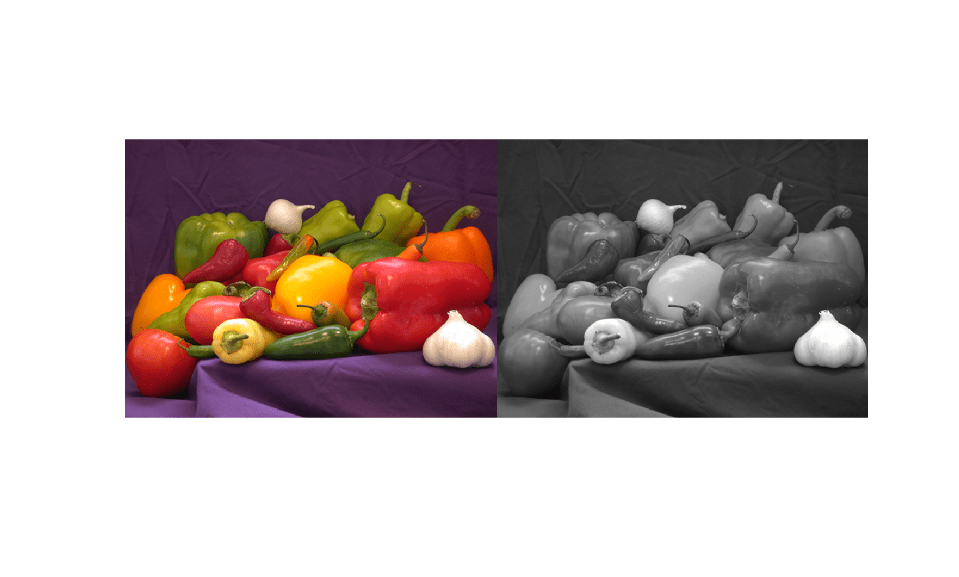Using an ROI, set the alpha layer (transparency) of two stacked images so that one image shows through only inside the ROI. This selective view follows the ROI so it can be moved interactively. Create a new figure and an axes.

```hFigure = figure(); hAxes = axes('Parent', hFigure); ```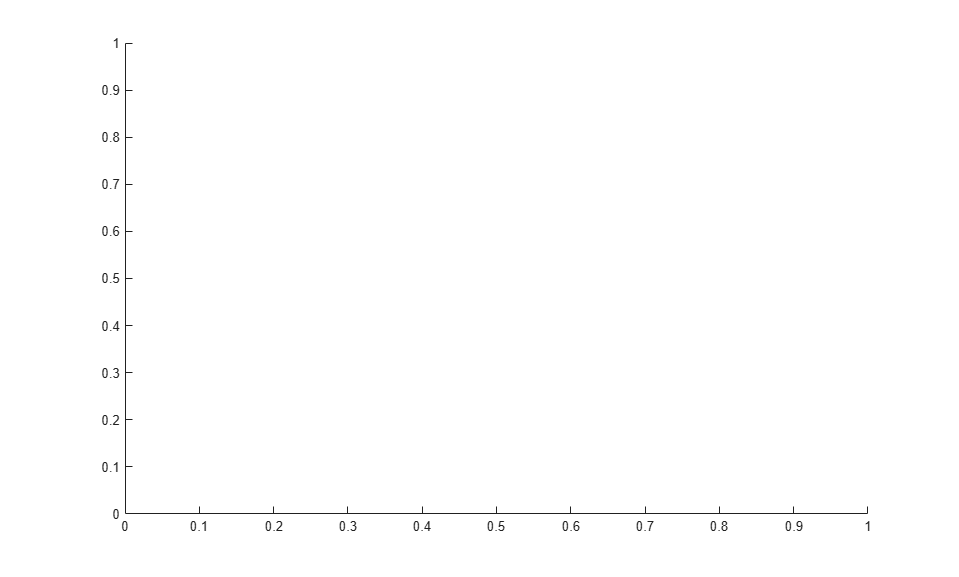Stack both images on the same axes.

```hImage1 = imshow(im, 'Parent', hAxes); drawnow; % Ensure the image gets drawn. hold on; hImage2 = imshow(imgray, 'Parent', hAxes); hold off; ```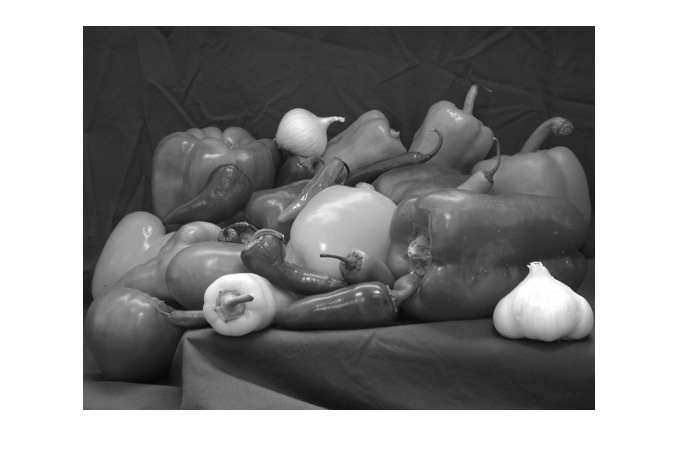Create a circular ROI on the axes.

```centerLocation = [220, 100]; radius = 60; hC = images.roi.Circle(... 'Parent', hAxes,... 'FaceAlpha',0,... 'Center',centerLocation,... 'Radius', radius); ```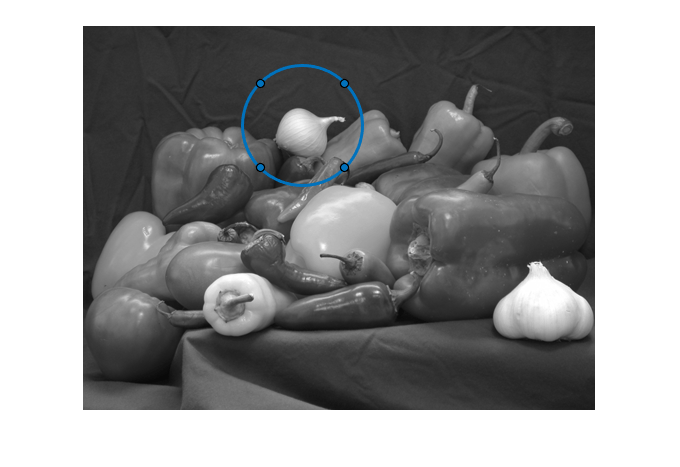Create a listener that listens to changes in the position of the ROI (the circle). The updateAlpha function is defined at the end of this example.

```addlistener(hC,'MovingROI', @updateAlpha); ```

Execute the callback manually the first time

```updateAlpha(hC) ```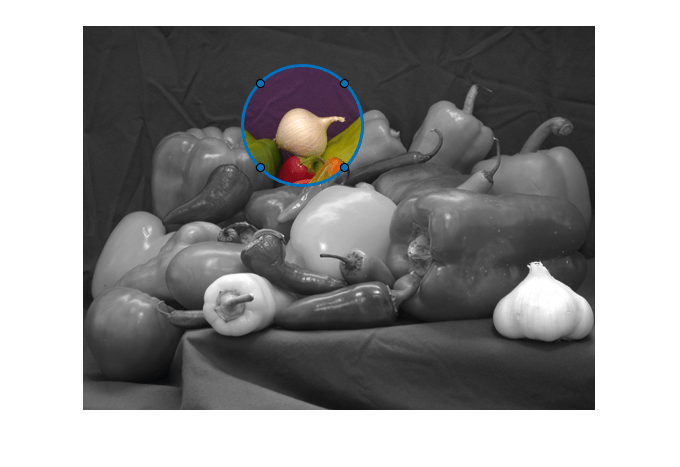Simulate zooming in to a region.

```hC.Parent.XLim = [75 370]; hC.Parent.YLim = [0 205]; ```This file contains the source code for a function that implements this image comparison tool. This code listens for two additional events. When a user to enter the 't/T' key to switch which image is on top. The code also listens for the mouse scroll wheel to increase or decrease the radius of the ROI.

```edit helperImageComparer ```

### An Animation of the Tool in Use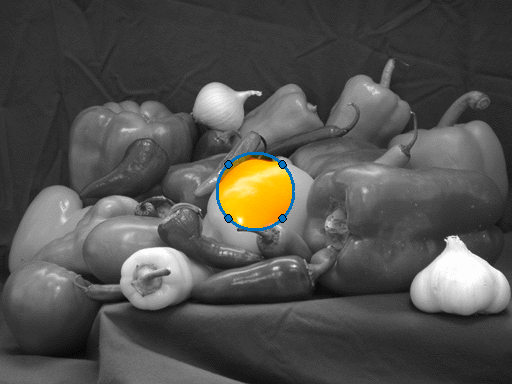### Callback Function to Update Alpha Layer

Callback function to update the alpha layer as the ROI object is moved.

```function updateAlpha(hC, ~) hImages = findobj(hC.Parent,'Type','image'); % Create a BW mask from the Circle ROI mask = hC.createMask(hImages(1).CData); % Set the alpha data so that the underlying image shows through % only inside the circle set(hImages(1),'AlphaData', ~mask); end ```

Download ebook